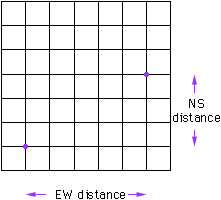Hi, Im doing an investigation entitled 'taxicab geometry', ive attempted it and have done most of the practical part of it. But i cant seem to see the equation behind it all. please guide me in the right direction. They provide a regular grid with regular horizontal and vertical lines. The lines are roads and the actual squares are blocks of hoouses/ buildings. In taxicab geometry the distance between place A & B is worked out by adding together the horizontal and vertical distances. each square is counted as i unit. Part 1 (I've done this) A taxix cab firm is based at A. the posistion B is 7 units away from A. investigate all the posistions of B at 7 units. investigate for different distances. I found that all the points when plotted form a diamond shape. the same shape is formd for different ammounts of units. what ormula can u get for the posistions of the points. I have allready found a formuls for the number of points there are. Part2 The bus station is X. The railway station is Y. The didtance XY=6 units. investigate to find where the taxicab firm could be sited, so that the same distance from the bus station and the railway staion. What does happen if the locations of the bus and the railwa station are varied??? Part 3 The taxi cab firm decides to site its office so that the distance from the office to the bus sation is twice the distance to the railwa station.Where could the office be sited? ALL the possible posistions and Formmula???? Please help, i havent left it to the last minute, but i know alread i don't understand!!! Desperatley Jack Hi Jack, The taxicab distance between two points is the sum of the East-West distance and the North-South distance. The East-West distance is the difference between the X-coordinates of the points and the North-South distance is the difference between the Y-coordinates. (In each case you need to ensure that you subtract the smaller from the larger so that the distance can't be negative.) Thus if two points have coordinates (a,b) and (c,d) then an algebraic expression for the taxicab distance between them is |a-c| + |b-d| Hence in the diagram below if the lower left corner is the origin then the taxicab distance between the points is |1-6| + |1-4| = 8.Cheers, Penny

Go to Math Central